How to Learn in 24 Hours?

 Need Help? M-F: 9am-5pm(PST): Toll-Free: (877) RAPID-10 or 1-877-727-4310 24/7 Online Technical Support: The Rapid Support Center vip@rapidlearningcenter.com Secure Online Order:Need Proof? Testimonials by Our Users

 Rapid Learning Courses: MCAT in 24 Hours (2021-22) USMLE in 24 Hours (Boards) Chemistry in 24 Hours Biology in 24 Hours Physics in 24 Hours Mathematics in 24 Hours Psychology in 24 Hours SAT in 24 Hours ACT in 24 Hours AP in 24 Hours CLEP in 24 Hours DAT in 24 Hours (Dental) OAT in 24 Hours (Optometry) PCAT in 24 Hours (Pharmacy) Nursing Entrance Exams Certification in 24 Hours eBook - Survival Kits Audiobooks (MP3)

 Tell-A-Friend: Have friends taking science and math courses too? Tell them about our rapid learning system.Home »  Mathematics »  College Algebra

Logarithmic Functions

 Topic Review on "Title": Logarithmic function: The inverse function of the exponential function is called the logarithmic function and is denoted as: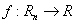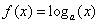where a is the base of the logarithm and it is must be >0 and not equal to one. Properties of logarithmic functions: 1) If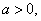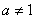and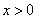then. 2) Ifthen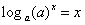. 3) If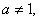then. 4) If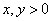then. 5) Ifandthen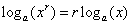.

Rapid Study Kit for "Title":
 Flash Movie Flash Game Flash Card Core Concept Tutorial Problem Solving Drill Review Cheat Sheet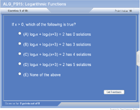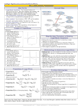"Title" Tutorial Summary : Logarithms are the inverse functions of exponentials as it is described in this tutorial.  The graphs of logarithms follow the same rules as the ones of exponentials except they are not defined at zero. The concept of one to one functions is used to verify that logarithms have inverses. Examples of logarithms are shown with the use of graphs. Properties of logarithms are similar to the ones of exponentials except that they are not defined at zero. Logarithms properties are used to solve expressions involving them and are presented in the examples. Application of logarithms in economics and finance are used in word problems.

 Tutorial Features: Specific Tutorial Features: • Examples to illustrate the properties and applications of logarithms. • Animated diagrams to actually show the inverse relationship between an exponential and a logarithm. • Problem-solving techniques are used to work out and illustrate the example problems, step by step. Series Features: • Concept map showing inter-connections of new concepts in this tutorial and those previously introduced. • Definition slides introduce terms as they are needed. • Visual representation of concepts • Animated examples—worked out step by step • A concise summary is given at the conclusion of the tutorial.

 "Title" Topic List: Preliminaries of logarithmsLogarithms functions and their properties Definition of logarithms Properties of logarithms Logarithmic equationsCommon and Natural Logarithms Euler Number Logarithms with different bases Application of logarithms

See all 24 lessons in College Algebra, including concept tutorials, problem drills and cheat sheets:
Teach Yourself College Algebra Visually in 24 Hours